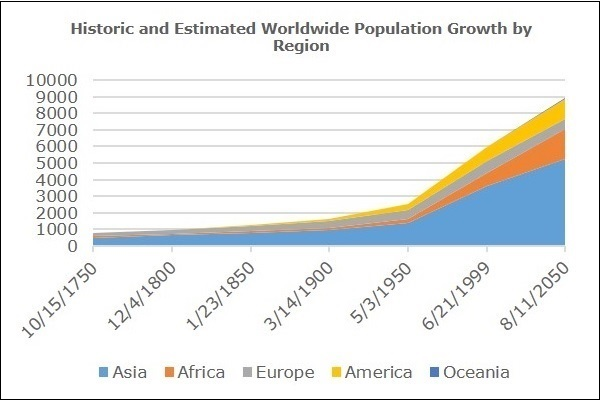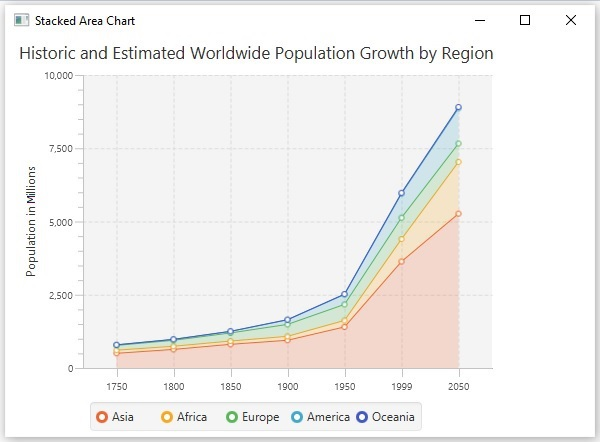# JavaFX - Stacked Area Chart

Advertisements

StackedArea Chart is a variation of the Area Chart that displays trends of the contribution of each value (For example – overtime). The areas are stacked so that each series adjoins, but does not overlap the preceding series. This contrasts with the Area chart where each series overlays the preceding series.

Following is a Stacked chart depicting population growth.In JavaFX, a Stacked Area chart is represented by a class named StackedAreaChart. This class belongs to the package javafx.scene.chart. By instantiating this class, you can create a StackedAreaChart node in JavaFX.

## Steps to Generate Stacked Area Chart

To generate a stacked area chart in JavaFX, follow the steps given below.

### Step 1: Creating a Class

Create a Java class and inherit the Application class of the package javafx.application. Then you can implement the start() method of this class as follows.

```public class ClassName extends Application {
@Override
public void start(Stage primaryStage) throws Exception {
}
}
```

### Step 2: Defining the Axis

Define the X and Y axis of the stacked area chart and set labels to them. In our example, X axis represents various years from 1750 to 2050. These have major tick units for every 50 years. While the Y axis represents the population growth in millions.

```//Defining the X axis
CategoryAxis xAxis = new CategoryAxis();

xAxis.setCategories(FXCollections.<String>observableArrayList
(Arrays.asList("1 750", "1800", "1850", "1900", "1950", "1999", "2050" )));

//Defining the Y axis
NumberAxis yAxis = new NumberAxis(0, 10000, 2500);
yAxis.setLabel("Population in Billions");
```

### Step 3: Creating the Stacked Area Chart

Create a line chart by instantiating the class named StackedAreaChart of the package javafx.scene.chart. To the constructor of this class, pass the objects representing the X and Y axis created in the previous step.

```//Creating the Area chart
StackedAreaChart<String, Number> areaChart = new StackedAreaChart(xAxis, yAxis);
areaChart.setTitle("Historic and Estimated Worldwide Population Growth by Region");
```

### Step 4: Preparing the Data

Instantiate the XYChart.Series class and add the data (a series of, x and y coordinates) to the Observable list of this class as follows −

```//Prepare XYChart.Series objects by setting data
XYChart.Series series1 = new XYChart.Series();
series1.setName("Asia");
series1.getData().add(new XYChart.Data("1750", 502));
series1.getData().add(new XYChart.Data("1800", 635));
series1.getData().add(new XYChart.Data("1850", 809));
series1.getData().add(new XYChart.Data("1900", 947));
series1.getData().add(new XYChart.Data("1950", 1402));
series1.getData().add(new XYChart.Data("1999", 3634));
series1.getData().add(new XYChart.Data("2050", 5268));

XYChart.Series series2 = new XYChart.Series();
series2.setName("Africa");
series2.getData().add(new XYChart.Data("1750", 106));
series2.getData().add(new XYChart.Data("1800", 107));
series2.getData().add(new XYChart.Data("1850", 111));
series2.getData().add(new XYChart.Data("1900", 133));
series2.getData().add(new XYChart.Data("1950", 221));
series2.getData().add(new XYChart.Data("1999", 767));
series2.getData().add(new XYChart.Data("2050", 1766));

XYChart.Series series3 = new XYChart.Series();
series3.setName("Europe");
series3.getData().add(new XYChart.Data("1750", 163));
series3.getData().add(new XYChart.Data("1800", 203));
series3.getData().add(new XYChart.Data("1850", 276));
series3.getData().add(new XYChart.Data("1900", 408));
series3.getData().add(new XYChart.Data("1950", 547));
series3.getData().add(new XYChart.Data("1999", 729));
series3.getData().add(new XYChart.Data("2050", 628));

XYChart.Series series4 = new XYChart.Series();
series4.setName("America");
series4.getData().add(new XYChart.Data("1750", 18));
series4.getData().add(new XYChart.Data("1800", 31));

series4.getData().add(new XYChart.Data("1850", 54));
series4.getData().add(new XYChart.Data("1900", 156));
series4.getData().add(new XYChart.Data("1950", 339));
series4.getData().add(new XYChart.Data("1999", 818));
series4.getData().add(new XYChart.Data("2050", 1201));

XYChart.Series series5 = new XYChart.Series();
series5.setName("Oceania");
series5.getData().add(new XYChart.Data("1750", 2));
series5.getData().add(new XYChart.Data("1800", 2));
series5.getData().add(new XYChart.Data("1850", 2));
series5.getData().add(new XYChart.Data("1900", 6));
series5.getData().add(new XYChart.Data("1950", 13));
series5.getData().add(new XYChart.Data("1999", 30));
series5.getData().add(new XYChart.Data("2050", 46));
```

### Step 5: Add Data to the Stacked Area Chart

Add the data series prepared in the previous step to the stacked area chart as follows −

```//Setting the data to area chart
areaChart.getData().addAll(series1, series2, series3, series4, series5);
```

### Step 6: Creating a Group Object

In the start() method, create a group object by instantiating the class named Group, which belongs to the package javafx.scene.

Pass the StackedAreaChart (node) object created in the previous step as a parameter to the constructor of the Group class. This should be done in order to add it to the group as follows −

```Group root = new Group(stackedAreaChart);
```

### Step 7: Creating a Scene Object

Create a Scene by instantiating the class named Scene, which belongs to the package javafx.scene. To this class, pass the Group object (root) created in the previous step.

In addition to the root object, you can also pass two double parameters representing height and width of the screen, along with the object of the Group class as follows.

```Scene scene = new Scene(group ,600, 300);
```

### Step 8: Setting the Title of the Stage

You can set the title to the stage using the setTitle() method of the Stage class. The primaryStage is a Stage object, which is passed to the start method of the scene class as a parameter.

Using the primaryStage object, set the title of the scene as Sample Application as follows.

```primaryStage.setTitle("Sample Application");
```

### Step 9: Adding Scene to the Stage

You can add a Scene object to the stage using the method setScene() of the class named Stage. Add the Scene object prepared in the previous steps using this method as follows.

```primaryStage.setScene(scene);
```

### Step 10: Displaying the Contents of the Stage

Display the contents of the scene using the method named show() of the Stage class as follows.

```primaryStage.show();
```

### Step 11: Launching the Application

Launch the JavaFX application by calling the static method launch() of the Application class from the main method as follows.

```public static void main(String args[]){
launch(args);
}
```

### Example

The following table lists out the population of different continents from year 1750 till year 2050.

Asia Africa Europe America Oceania 502 106 163 18 2 635 107 203 31 2 809 111 276 54 2 947 133 408 156 6 1402 221 547 339 13 3634 767 729 818 30 5268 1766 628 1201 46

Following is a Java program which generates a stacked area chart depicting the above data using JavaFX.

Save this code in a file with the name StackedAreaChartExample.java.

```import java.util.Arrays;
import javafx.application.Application;
import static javafx.application.Application.launch;
import javafx.collections.FXCollections;
import javafx.scene.Group;
import javafx.scene.Scene;
import javafx.scene.chart.CategoryAxis;
import javafx.stage.Stage;
import javafx.scene.chart.NumberAxis;
import javafx.scene.chart.StackedAreaChart;
import javafx.scene.chart.XYChart;

public class StackedAreaChartExample extends Application {
@Override
public void start(Stage stage) {
//Defining the axes
CategoryAxis xAxis = new CategoryAxis();
xAxis.setCategories(FXCollections.<String>observableArrayList(
Arrays.asList("1750", "1800", "1850", "1900", "1950", "1999", "2050" )));

NumberAxis yAxis = new NumberAxis(0, 10000, 2500);
yAxis.setLabel("Population in Millions");

//Creating the Area chart
StackedAreaChart<String, Number> areaChart = new StackedAreaChart(xAxis, yAxis);
areaChart.setTitle("Historic and Estimated Worldwide Population Growth by Region");

//Prepare XYChart.Series objects by setting data
XYChart.Series series1 = new XYChart.Series();

series1.setName("Asia");
series1.getData().add(new XYChart.Data("1750", 502));
series1.getData().add(new XYChart.Data("1800", 635));
series1.getData().add(new XYChart.Data("1850", 809));
series1.getData().add(new XYChart.Data("1900", 947));
series1.getData().add(new XYChart.Data("1950", 1402));
series1.getData().add(new XYChart.Data("1999", 3634));
series1.getData().add(new XYChart.Data("2050", 5268));

XYChart.Series series2 = new XYChart.Series();
series2.setName("Africa");
series2.getData().add(new XYChart.Data("1750", 106));
series2.getData().add(new XYChart.Data("1800", 107));
series2.getData().add(new XYChart.Data("1850", 111));
series2.getData().add(new XYChart.Data("1900", 133));
series2.getData().add(new XYChart.Data("1950", 221));
series2.getData().add(new XYChart.Data("1999", 767));
series2.getData().add(new XYChart.Data("2050", 1766));

XYChart.Series series3 = new XYChart.Series();
series3.setName("Europe");

series3.getData().add(new XYChart.Data("1750", 163));
series3.getData().add(new XYChart.Data("1800", 203));
series3.getData().add(new XYChart.Data("1850", 276));
series3.getData().add(new XYChart.Data("1900", 408));
series3.getData().add(new XYChart.Data("1950", 547));
series3.getData().add(new XYChart.Data("1999", 729));
series3.getData().add(new XYChart.Data("2050", 628));

XYChart.Series series4 = new XYChart.Series();
series4.setName("America");
series4.getData().add(new XYChart.Data("1750", 18));
series4.getData().add(new XYChart.Data("1800", 31));
series4.getData().add(new XYChart.Data("1850", 54));
series4.getData().add(new XYChart.Data("1900", 156));
series4.getData().add(new XYChart.Data("1950", 339));
series4.getData().add(new XYChart.Data("1999", 818));
series4.getData().add(new XYChart.Data("2050", 1201));

XYChart.Series series5 = new XYChart.Series();
series5.setName("Oceania");
series5.getData().add(new XYChart.Data("1750", 2));
series5.getData().add(new XYChart.Data("1800", 2));
series5.getData().add(new XYChart.Data("1850", 2));
series5.getData().add(new XYChart.Data("1900", 6));
series5.getData().add(new XYChart.Data("1950", 13));
series5.getData().add(new XYChart.Data("1999", 30));
series5.getData().add(new XYChart.Data("2050", 46));

//Setting the data to area chart
areaChart.getData().addAll(series1, series2, series3, series4, series5);

//Creating a Group object
Group root = new Group(areaChart);

//Creating a scene object
Scene scene = new Scene(root, 600, 400);

//Setting title to the Stage
stage.setTitle("Stacked Area Chart");

//Adding scene to the stage
stage.setScene(scene);

//Displaying the contents of the stage
stage.show();
}
public static void main(String args[]){
launch(args);
}
}
```

Compile and execute the saved java file from the command prompt using the following commands.

```javac StackedAreaChartExample.java
java StackedAreaChartExample
```

On executing, the above program generates a JavaFX window displaying a stacked area chart as shown below.javafx_charts.htm
Advertisements